### 1.区间合并

1. M a b，将编号为 ab 的两个数所在的集合合并，如果两个数已经在同一个集合中，则忽略这个操作；
2. Q a b，询问编号为 ab 的两个数是否在同一个集合中；

#### 数据范围

$1≤n,m≤10^5$

#### 输入样例：

4 5
M 1 2
M 3 4
Q 1 2
Q 1 3
Q 3 4

#### 输出样例：

Yes
No
Yes

### 2.思路

#### 初始化

for(int i = 1; i <= n; i ++) p[i] = i;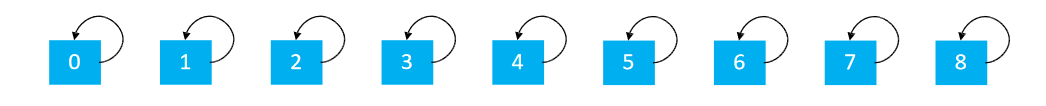#### 查找 + 路径压缩

int find(int x){ //返回x的祖先节点 + 路径压缩
//祖先节点的父节点是自己本身
if(p[x] != x){
//将x的父亲置为x父亲的祖先节点,实现路径的压缩
p[x] = find(p[x]);
}
return p[x];
}

find的功能是用于查找祖先节点，那么路径压缩又是怎么完成的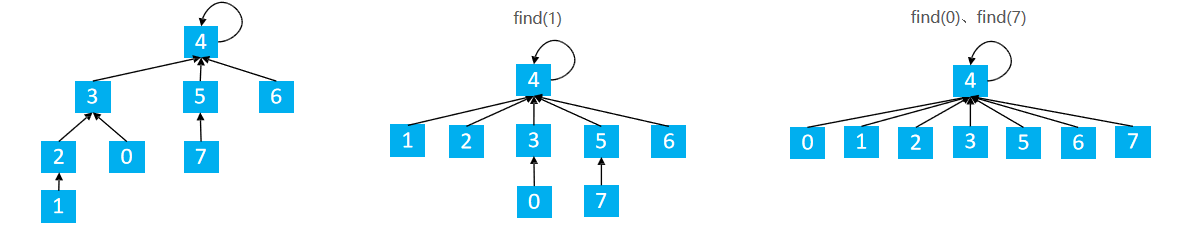find(1) p = 2 p = find(2)
find(2) p = 3 p = find(3)
find(3) p = 4 p = find(4)
find(4) p = 4 将p返回

find(3) p = 4 p = 4 将p返回

find(2) p = 3 p = 4 将p返回

find(1) p = 2 p = 4 将p返回

nice!!

#### 合并操作

if(op == 'M') p[find(a)] = find(b); //将a的祖先点的父节点置为b的祖先节点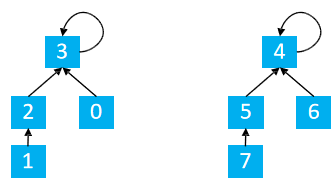find(1) = 3 find(5) = 4
p[find(1)] = find(5) –> p = 4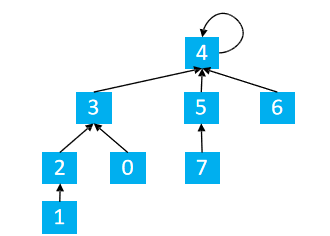#### 查找

if (find(a) == find(b)) puts("在同一个集合中");
else puts("不在同一个集合");

#### 其他路径压缩方法

1. 路径分裂：使路径上的每个节点都指向其祖父节点（parent的parent）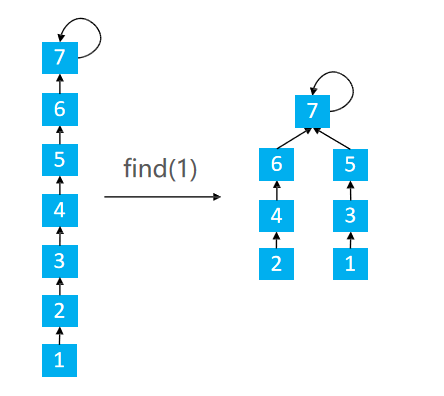int find(int x){
while(x != p[x]){
int parent = p[x];
p[x] = p[p[x]];
x = parent;
}
return x;
}
1. 路径减半：使路径上每隔一个节点就指向其祖父节点（parent的parent）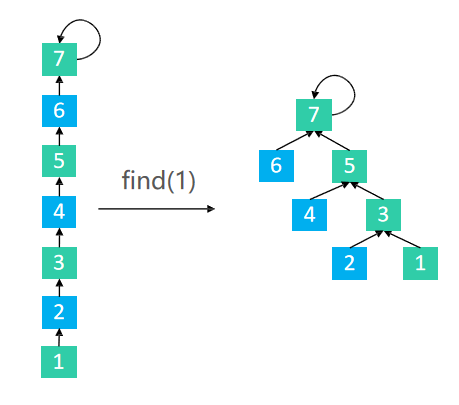int find(int x){
while(x != p[x]){
p[x] = p[p[x]];
x = p[x];
}
return x;
}

### 3.总结

1. 将两个集合合并
2. 询问两个元素是否在一个集合中

1. 判断树根 if(p[x] = x)
2. 求x的集合编号 while(p[x] != x) x = p[x]
3. 合并两个集合，这两将x的根节点嫁接到y的根节点, pxx的根节点， pyy的根节点，嫁接p[px] = py

### 4.C++代码

#include <iostream>
using namespace std;

const int N = 1e5 + 10;
int p[N];
int n, m;

void init() {
for (int i = 1; i <= n; i ++) p[i] = i;
}

int find(int x) {   // 返回x的祖宗节点 + 路径压缩
if (p[x] != x) p[x] = find(p[x]);
return p[x];
}

int main() {
cin >> n >> m;
init();
while (m --) {
char op;
int a, b;
cin >> op >> a >> b;

if (op == 'M') {
p[find(a)] = find(b);    // a的祖宗节点的父节点等于b的祖宗节点
} else {
if (find(a) == find(b)) puts("Yes");
else puts("No");
}
}

return 0;
}

### 4.变形

#### 连通块中点的数量

1. C a b，在点 a 和点 b 之间连一条边，ab 可能相等；
2. Q1 a b，询问点 a 和点 b 是否在同一个连通块中，ab 可能相等；
3. Q2 a，询问点 a 所在连通块中点的数量；

#### 数据范围

$1≤n,m≤10^5$

#### 输入样例：

5 5
C 1 2
Q1 1 2
Q2 1
C 2 5
Q2 5

#### 输出样例：

Yes
2
3

### 5.分析

for(int i = 1; i <= n; i ++) {
p[i] = i;
//用祖先节点记录当前合并集合的size
size[i] = 1;
}find(1) = 3 find(5) = 4
p = 4

size = 4 以3为根节点下有4个连通块
size = 4 以4为根节点下有4个连通块

size = size + size = 8

### C++代码

#include <iostream>
using namespace std;

const int N = 1e5 + 10;
int n, m;
int p[N], cnt[N];

void init() {
for (int i = 1; i <= n; i ++) {
p[i] = i;
cnt[i] = 1;
}
}

int find(int x) {
if (p[x] != x) p[x] = find(p[x]);
return p[x];
}

int main() {
cin >> n >> m;
init();

string op;
int a, b;

while (m --) {
cin >> op;
if (op == "C") {
cin >> a >> b;
a = find(a), b = find(b);
if (a != b) {
p[a] = b;
cnt[b] += cnt[a];
}
} else if (op == "Q1") {
cin >> a >> b;
if (find(a) == find(b)) puts("Yes");
else puts("No");
} else {
cin >> a;
cout << cnt[find(a)] << endl;
}
}

return 0;
}